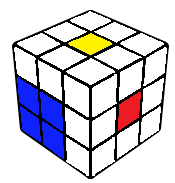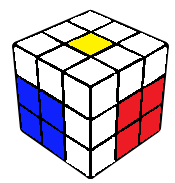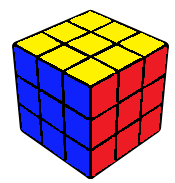# YruRU Method### EO-CP-2x2x3

This is where one is expected to end up after successfully doing the first three steps. With the R and U layers remaining, edges oriented and corners permuted, the rest of the cube can be solved using only R and U moves. This is typically done as F2L followed by LL.### Square

A 2x2x1 block is constructed on the first two layers. This can be done by connecting one edge with the right centre, followed by the solving of a corner-edge pair. The edge can usually be connected such that we get an easy corner-edge pair case.### F2L

The final corner edge pair is solved, typically intuitively. However, this can also be broken down into 20 cases. This is less than the typical CFOP last slot since EO is already done. The algorithms for each case can be found in the F2L algorithms section.### 2GLL

Since CP and EO are already done, the last layer can be done using one of only 84 algorithms, all of which are 2-gen. Every case and beginners' recognition techniques are listed in the 2GLL section. Different ways to finish can be found in the advanced section.

Average movecount: ~ 14

Average double moves: ~ 4

Algorithms: 20

If no F2L block is preserved at the end of the EOBF step, the square can be constructed using any F2L edge, connected with an adjacent corner edge pair. The sole edge needs to be attached to the right centre and placed down such that the following corner edge pair is set up to a good case, with a possibility of cancellations into insertion of the pair. There isn't exactly a practical algorithmic way to do this, experience and analysis of examples will over time build the repertoire required to handle each case almost optimally. The general rule of thumb is, 3 move inserts are better than split pairs in top layer, which are in turn better than incorrectly connected pairs in the top layer, which are better than pairs with one piece not in the top layer, and so on.